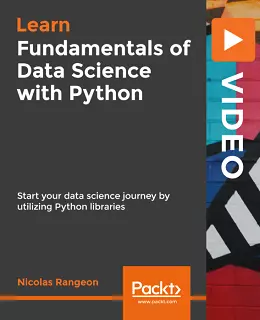# Fundamentals of Data Science with Python [Video]### Fundamentals of Data Science with Python [Video]

##### English | MP4 | AVC 1920×1080 | AAC 48KHz 2ch | 2h 38m | 437 MB eLearning | Skill level: All Levels

Fundamentals of Data Science with Python [Video]: Implement powerful data science techniques with Python using NumPy, SciPy, Matplotlib, and scikit-learn

Python has grown into a key language that can be used to develop solutions for a variety of data science challenges. This course will teach you the fundamentals of data science using Python and its growing collection of libraries that focus on particular elements of data science.

In this course, we will get hands-on with a variety of data science tasks. After a quick primer on Python, you will start with a quick task: sourcing, processing, and cleaning a dataset. Then, you will use Python to mine data from its source and analyze available data via statistical and probability analysis techniques by using NumPy and pandas. You will also look at modeling data in order to perform Artificial Intelligence prediction by using the SciPy, scikit-learn, and statsmodels libraries. The course also covers visualization methods using the Matplotlib library to display this analysis and visually demonstrate patterns in the data.

• Understand simple statistics and probability using NumPy
• Work with Bayesian statistical analysis with NumPy library
• Perform statistical modeling and fitting using the NumPy, SciPy, and statsmodels libraries
• Use Python’s graphics libraries to plot data with the Matplotlib library
• Work with the scikit-learn library to build AI models

By the end of this course, you will be able to work on data science tasks in a practical way with different Python libraries and achieve your goals.

### 1 Response

1. March 4, 2020

[…] of Statistics and Visualization in Python […]